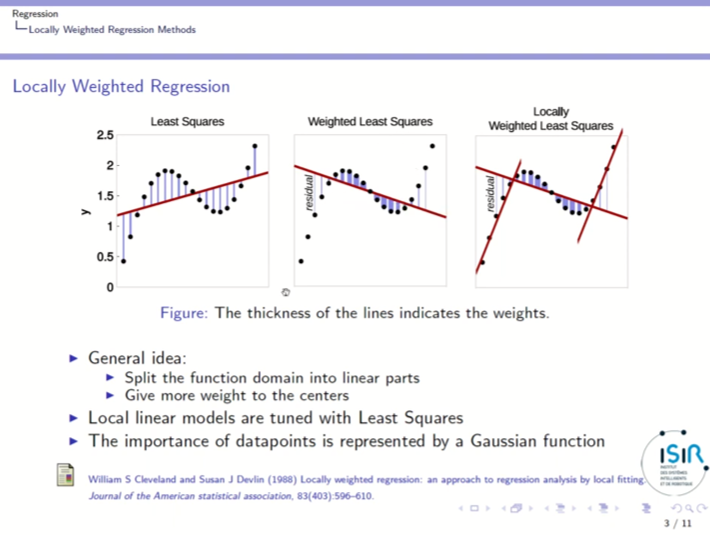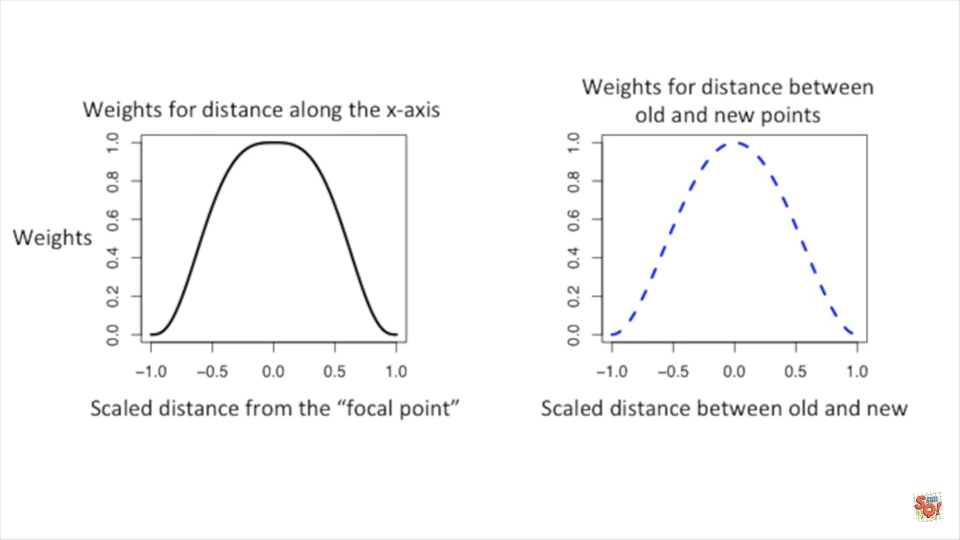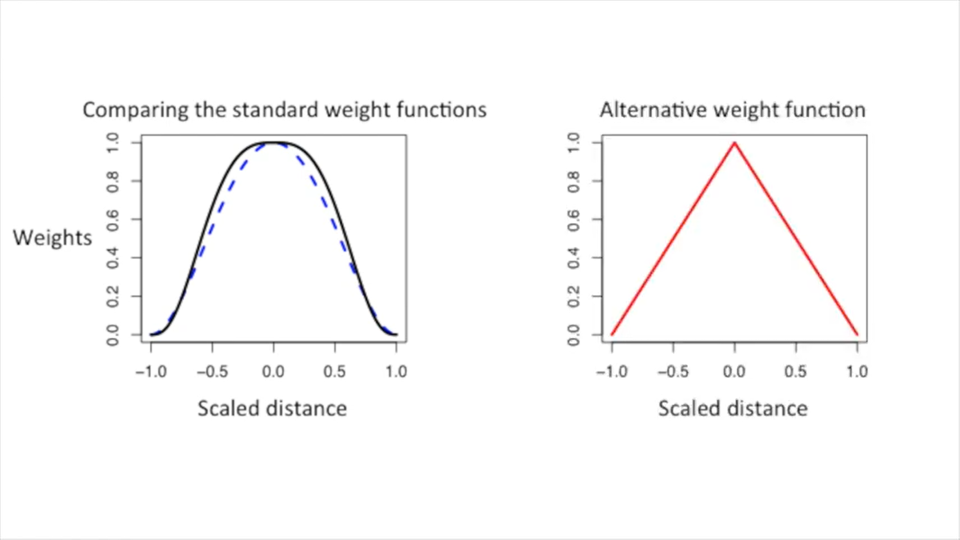LOWESS Smoothing
Post
Cancel

# LOWESS Smoothing

## Basic Information

Local regression or local polynomial regression, also known as moving regression, is a generalization of moving average and polynomial regression. Its most common methods, initially developed for scatterplot smoothing, are LOESS (locally estimated scatterplot smoothing) and LOWESS (locally weighted scatterplot smoothing). From wikipedia

## Introduction

• Least Squares finds the line with the minimal squared distance from the data points.
• But how to fit a curve to data?
• LOWESS Smmothing use least squares to do it.## Idea

• Use a type of sliding window to diveide the data into smaller blobs
• At each data point, use a type of least squares(weighted) to fit a line
• To reduce the influence on the new curve, we create an additional weight for the weighted least squares based on how far the original point is from the new point
• Iter

## Weight Functions

 Different weight functions Comparison of weight functions## Code Example

https://github.com/StatQuest/lowess_loess_demo/blob/master/lowess_loess_demo.R

1 2 3 4 5 6 7 8 9 10 11 12 13 14 15 16 17 18 19 20 21 22 23 24 25 26 27 28 29 30 31 32 33 34 35 36 37 38 39 40 41 42 43 44 45 46 47 48 49 50 51 52 ## first let's make a noisy gamma distribution plot... x <- seq(from=0, to=20, by=0.1) y.gamma <- dgamma(x, shape=2, scale=2) y.gamma.scaled <- y.gamma * 100 y.norm <- vector(length=201) for (i in 1:201) { y.norm[i] <- rnorm(n=1, mean=y.gamma.scaled[i], sd=2) } data <- data.frame(x, y.norm) plot(data, frame.plot=FALSE, xlab="", ylab="", col="#d95f0e", lwd=1.5) ## Now that we have the data, let's look at the differences ## and similarities between R's lowess() function and the loess() function. ## We'll start with the lowess() function... ## ## By default "lowess()" fits a line in each window using ## 2/3's of the data points. ## ## the first parameter, y.norm ~ x, says that y.norm is being ## modeled by x, and the second parameter, f, is the fraction ## of points to use in each window. Here, we're using 1/5 of the ## data points in each window. lo.fit.gamma <- lowess(y.norm ~ x, f=1/5) plot(data, frame.plot=FALSE, xlab="", ylab="", col="#d95f0e", lwd=1.5) lines(x, lo.fit.gamma$y, col="black", lwd=3) ## Now use loess() to fit a curve to the data... ## ## By default "loess()" fits a parabola in each window using ## 75% of the data points. plx<-predict(loess(y.norm ~ x, span=1/5, degree=2, family="symmetric", iterations=4), se=T) plot(data, frame.plot=FALSE, xlab="", ylab="", col="#d95f0e", lwd=1.5) lines(x, plx$fit, col="black", lwd=3) ## Now let's add a confidence interval to the loess() fit... plot(data, type="n", frame.plot=FALSE, xlab="", ylab="", col="#d95f0e", lwd=1.5) polygon(c(x, rev(x)), c(plx$fit + qt(0.975,plx$df)*plx$se, rev(plx$fit - qt(0.975,plx$df)*plx$se)), col="#99999977") points(data, col="#d95f0e", lwd=1.5) lines(x, plx\$fit, col="black", lwd=3) ## Now that we know how those functions work... we can skip all that ## nasty stuff and just use ggplot2 with geom_point() and geom_smooth() library(ggplot2) ggplot(data=data, aes(x=x, y=y.norm)) + geom_point() + geom_smooth(span=1/5)